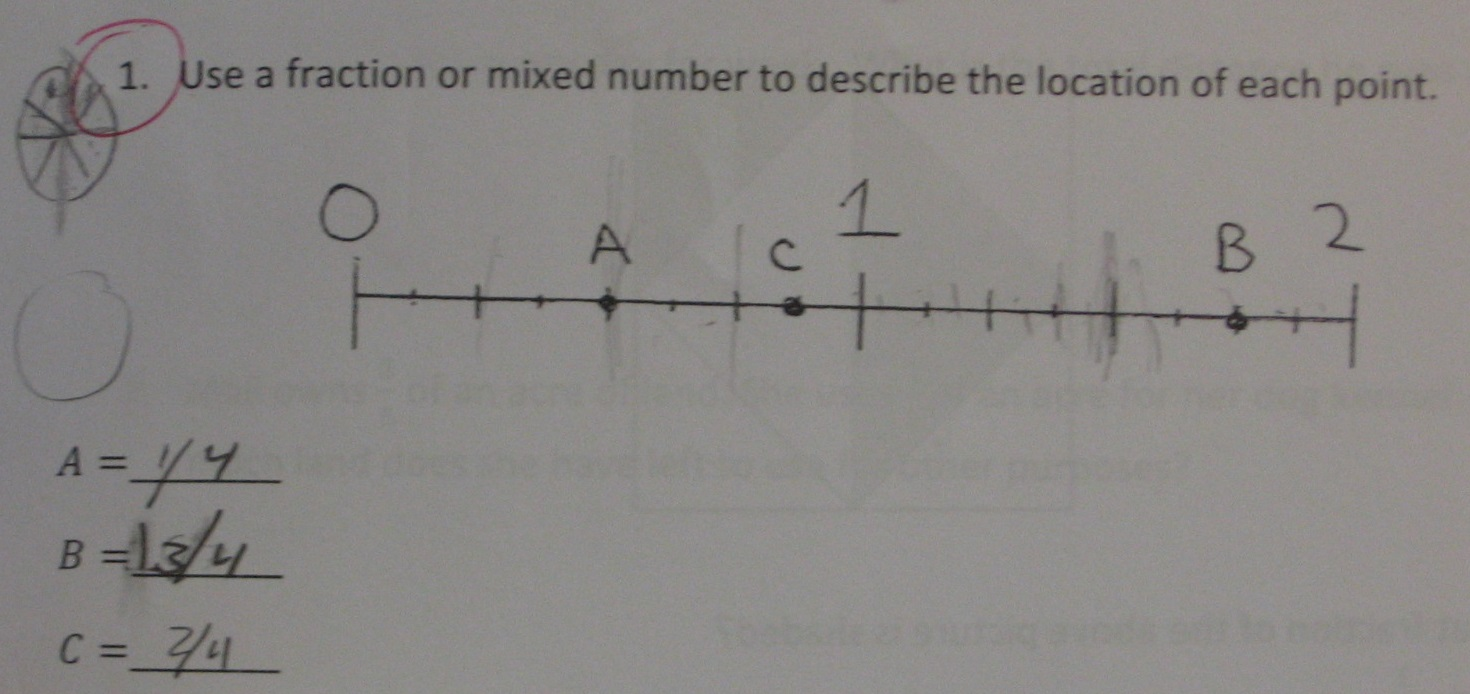Categories

# Fractions Mistakes In The WildOne of the joys of teaching fractions for the first time is seeing mistakes from my students that, prior to now, I’ve only read about.

(Does that make me a dork?)

(Yes, Michael, that makes you a dork.)

Anyway, the first mistake is an absolute classic. With numbers that show any sort of structural complexity, students regularly treat the components individually. See, decimals, complex numbers, polynomials, and other things I’m sure. (If you have links or suggestions of other sorts of common mistakes that fit this paradigm, drop a comment in the comment hole.)

The second mistake pushes on their part-whole understanding in a direct way. I’m not sure how this mistake plays itself out beyond the geometric context. Thoughts, people?

The third mistake is an interesting one, and one that I suspect is about being used to seeing number lines only of unit length before. Other than that, I don’t have much interesting to say about that. But maybe you do?

## 2 replies on “Fractions Mistakes In The Wild”

kmorrowleongsays:

The number line is interesting to me because of everything you said, and because the distance from C to 0 is not double the distance from A to 0! Since it seems as if the student is alternating his/her interpretation of the referent unit for each point, I would open the questioning for each point in turn with “What is the whole?” while covering the answers they put for the other two points. And following that answer, ask them to show 2/4 and then 1 3/4. This also makes me want to have a measuring activity the next day!
The rope problem is no different than the student who writes 96 – 68 as 32. 8 and 6 with a subtraction sign must be 2 and 90 – 60 is 30. 32. And in the same sense that the fractions are structurally complex, the base-10 numbers 96 and 68 are also complex structures. An open number line goes a long way to resolve these kinds of problems in a subtraction problem, as well as in a fraction subtraction problem. 68 to 70 is 2. 70 to 90 is 20. And 90 to 96 is 6. 2 + 20 + 6 = 28. This structure is similar to 3 3/8 to 4 being 5/8. 4 to 10 then is 6. 6 5/8. Much easier.

Laura C.says:

The second mistake happens because some students either forget that fractions are equally-sized parts of the whole, or assume that all the pieces must be equal because they’re in a math book picture, and math-class diagrams are just magical that way. The idea that the central square is 1/2 of the original square (and therefore, the left-hand colored section is 1/4)is also not obvious to a lot of students.

Not understanding the parts-of-the-whole idea of fractions makes it much harder to understand addition and subtraction of fractions. I’ve had so many remedial students to say that 4/5 – 1/3 = 3/2 and the like, because they can’t remember how to find a common denominator or why we need one for the problem. If I draw pies with 4/5 and 1/3 shaded in, it helps them to see that the answer must be less than 1–but even this isn’t a guarantee that they remember what to do!

As kmorrowleong said, problem 3 is a case of “I don’t know what the whole is.” If you have access to Cuisenaire rods, they’re easy to adapt into activities. “If the black rod is 1 whole, then how much is a red rod? An orange rod?” etc.